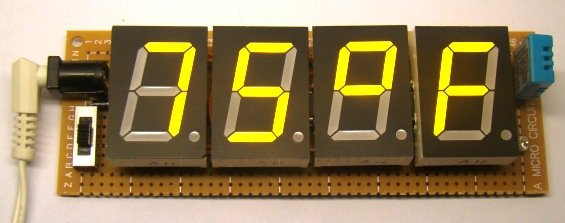# Current and Voltage Sense with Power Measurement

#### Description

The MAX44299 is a low-side current, voltage, and power monitoring circuit that provides an analog output current proportional to the measured current, voltage, and the internally calculated instantaneous power.

Instantaneous power is calculated internally by multiplying the load current and a fraction of the load voltage set by an external resistive divider. All three outputs are scaled to a full-scale current of 100µA. An additional output current of 100µA is available at the reference (REF) output; this current can be used to create a reference voltage for the ADC that is being used to measure the power, voltage, and current signals.By providing the ADC with both the measured signals and the input reference voltage, the ADC can make a ratiometric measurement, allowing improved accuracy. The use of currents, rather than voltage, to convey the measured signals to the ADC eliminates any errors caused by voltage drops across the parasitic resistance of PCB, which can be significant for high-current systems. To allow full-system calibration, the CAL bump provides a way to calibrate gain and offset for the ADC.

The device measures load current by using a precision, auto-zeroed current-sense amplifier (CSA), which due to its ultra-low offset voltage allows precise measurement of full-scale voltages of 5mV, 10mV, and 20mV. The load voltage is measured via a user-selectable resistive network, dividing the input voltage down to a full scale of 1.00V.

The wide supply voltage range of 3V to 5.5V allows the simple sharing of supplies with either the ADC or a microcontroller. The device can be powered down and the outputs will then go high impedance. The device is available in a 2.4mm x 2.4mm, 16-bump wafer-level package (WLP) and is specified for the 0°C to +85°C temperature range.

READ  RESEARCHERS FROM NREL DISCOVERED NEW METHOD TO DEVELOP RECHARGEABLE MAGNESIUM-METAL BATTERY

See the MAX44298 for a very similar product with a choice of 50µA output current and alternate pinout.

#### Key Features

• High Accuracy Improves Measurement Quality
• Accurate Power Measurement: < 1.1% of Reading Total Error
• Zero Thermal Drift Current-Sense Input
• High Integration Saves Cost and Space
• Power, Current, and Voltage Monitoring Plus Reference
• 5mV, 10mV, and 20mV Programmable Current-Sensing Full-Scale Voltage
• Calibration Point at 10μA upon Command

For more detail: Current and Voltage Sense with Power Measurement